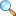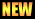# Unit 05: Sets and Functions

On this page the solutions and things related to Unit 05: Sets and Functions of Mathematics 10 (Science Group) (Matric) are given. These notes are send by Mr. Amir Shehzad, we are very thankful to him for sending these notes.

In this unit students will learn how to

• sets
• recall the sets denoted by $\mathbb{N}$, $\mathbb{W}$, $\mathbb{Z}$, $E$, $O$, $P$ and $\mathbb{Q}$.
• recognize operation on sets ($\cup$, $\cap$, $\setminus$,…)
• perform operations in set union, intersection, difference, complement.
• give formal proofs of the following fundamental properties of union and intersection of two or three sets
• commutative property of union.
• commutative property of intersection.
• associative property of union.
• associative property of intersection.

### Solutions

• Exercise 5.1 |View Online | Download PDF (116.35 KiB, 5081 downloads)
• Exercise 5.2 |View Online | Download PDF (65.15 KiB, 1580 downloads)• Exercise 5.4 |View Online | Download PDF (486.39 KiB, 7365 downloads)
• matric/10th_science/unit05
• Last modified: 2 months ago
• by Administrator